Appendix C Glossary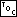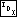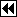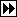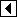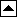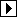adjacent: Two nodes in a binary tree (see binary tree) are adjacent if one is the child of the other.

AVL tree: A type of balanced tree (see balanced tree), where the AVL balance factor (see balance factor) of each node is limited to -1, 0, or +1.

balance: To rearrange a binary search tree (see binary search tree) so that it has its minimum possible height (see height), approximately the binary logarithm of its number of nodes.

balance condition: In a balanced tree (see balanced tree), the additional rule or rules that limit the tree's height.

balance factor: For any node in an AVL tree (see AVL tree), the difference between the height (see height) of the node's right subtree (see right subtree) and left subtree (see left subtree).

balanced tree: A binary search tree (see binary search tree) along with a rule that limits the tree's height in order to avoid a pathological case (see pathological case). Types of balanced trees: AVL tree (see AVL tree), red-black tree (see red-black tree).

binary search: A technique for searching by comparison of keys, in which the search space roughly halves in size after each comparison step.

binary search tree: A binary tree (see binary tree) with the additional property that the key in each node's left child is less than the node's key, and that the key in each node's right child is greater than the node's key. In inorder traversal (see inorder traversal), the items in a BST are visited in sorted order of their keys.

binary tree: A data structure that is either an empty tree (see empty tree) or consists of a root (see root), a left subtree (see left subtree), and a right subtree (see right subtree).

black box: Conceptually, a device whose input and output are defined but whose principles of internal operation is not specified.

black-height: In a red-black tree (see red-black tree), the number of black nodes along a simple path from a given node down to a non-branching node. Due to rule 2 (see rule 2), this is the same regardless of the path chosen.

BST: See binary search tree (see binary search tree).

child: In a binary tree (see binary tree), a left child (see left child) or right child (see right child) of a node.

children: More than one child (see child).

color: In a red-black tree (see red-black tree), a property of a node, either red or black. Node colors in a red-black tree are constrained by rule 1 (see rule 1) and rule 2 (see rule 2)

complete binary tree: A binary tree (see binary tree) in which every simple path (see simple path) from the root down to a leaf has the same length and every non-leaf node has two children.

compression: A transformation on a binary search tree used to rebalance (see rebalance) (sense 2).

deep copy: In making a copy of a complex data structure, it is often possible to copy upper levels of data without copying lower levels. If all levels are copied nonetheless, it is a deep copy. See also shallow copy (see shallow copy).

dynamic: 1. When speaking of data, data that can change or (in some contexts) varies quickly. 2. In C, memory allocation with malloc() and related functions. See also static (see static).

empty tree: A binary tree without any nodes.

height: In a binary tree, the maximum number of nodes that can be visited starting at the tree's root and moving only downward. An an empty tree has height 0.

idempotent: Having the same effect as if used only once, even if used multiple times. C header files are usually designed to be idempotent.

inorder predecessor: The node preceding a given node in an inorder traversal (see inorder traversal).

inorder successor: The node following a given node in an inorder traversal (see inorder traversal).

inorder traversal: A type of binary tree traversal (see traversal) where the root's left subtree is traversed, then the root is visited, then the root's right subtree is traversed.

iteration: In C, repeating a sequence of statements without using recursive function calls, most typically accomplished using a for or while loop. Oppose recursion (see recursion).

key: In a binary search tree, data stored in a node (see node) and used to order nodes.

leaf: A node (see node) whose children (see children) are empty.

left child: In a binary tree (see binary tree), the root of a node's left subtree, if that subtree is non-empty. A node that has an empty left subtree may be said to have no left child.

left rotation: See rotation.

left subtree: Part of a non-empty binary tree (see binary tree).

left-threaded tree: A binary search tree (see binary search tree) augmented to simplify and speed up traversal in reverse of inorder traversal (see inorder traversal), but not traversal in the forward direction.

literate programming: A philosophy of programming that regards software as a type of literature, popularized by Donald Knuth through his works such as [Knuth 1992].

node: The basic element of a binary tree, consisting of a key (see key), a left child (see left child), and a right child (see right child).

non-branching node: A node in a binary tree (see binary tree) that has exactly zero or one non-empty children.

nonterminal node: A node (see node) with at least one nonempty subtree (see subtree).

parent: When one node in a binary tree (see binary tree) is the child of another, the first node. A node that is not the child of any other node has no parent.

parent pointer: A pointer within a node to its parent (see parent) node.

pathological case: In a binary search tree (see binary search tree) context, a BST whose height (see height) is much greater than the minimum possible. Avoidable through use of balanced tree (see balanced tree) techniques.

path: In a binary tree (see binary tree), a list of nodes such that, for each pair of nodes appearing adjacent in the list, one of the nodes is the parent of the other.

postorder traversal: A type of binary tree traversal (see traversal) where the root's left subtree is traversed, then the root's right subtree is traversed, then the root is visited.

preorder traversal: A type of binary tree traversal (see traversal) where the root is visited, then the root's left subtree is traversed, then the root's right subtree is traversed.

rebalance: 1. After an operation that modifies a balanced tree (see balanced tree), to restore the tree's balance condition (see balance condition), typically by rotation (see rotation) or, in a red-black tree (see red-black tree), changing the color (see color) of one or more nodes. 2. To reorganize a binary search tree (see binary search tree) so that its shape more closely approximates that of a complete binary tree (see complete binary tree).

recursion: In C, describes a function that calls itself directly or indirectly. See also tail recursion (see tail recursion). Oppose iteration (see iteration).

red-black tree: A form of balanced tree (see balanced tree) where each node has a color (see color) and these colors are laid out such that they satisfy rule 1 (see rule 1) and rule 2 (see rule 2) for red-black trees.

right child: In a binary tree (see binary tree), the root of a node's right subtree, if that subtree is non-empty. A node that has an empty right subtree may be said to have no right child.

right rotation: See rotation.

right subtree: Part of a non-empty binary tree (see binary tree).

right-threaded tree: A binary search tree (see binary search tree) augmented to simplify and speed up inorder traversal (see inorder traversal), but not traversal in the reverse order.

rotation: A particular type of simple transformation on a binary search tree (see binary search tree) that changes local structure without changing inorder traversal (see inorder traversal) ordering. See BST Rotations, TBST Rotations, RTBST Rotations, and PBST Rotations, for more details.

root: A node (see node) taken as a binary tree (see binary tree) in its own right. Every node is the root of a binary tree, but “root” is most often used to refer to a node that is not a child (see child) of any other node.

rule 1: One of the rules governing layout of node colors in a red-black tree (see red-black tree): no red node may have a red child. See RB Balancing Rule.

rule 2: One of the rules governing layout of node colors in a red-black tree (see red-black tree): every simple path (see simple path) from a given node to one of its non-branching node (see non-branching node) descendants contains the same number of black nodes. See RB Balancing Rule.

sentinel: In the context of searching in a data structure, a piece of data used to avoid an explicit test for a null pointer, the end of an array, etc., typically by setting its value to that of the looked-for data item.

sequential search: A technique for searching by comparison of keys, in which the search space is typically reduced only by one item for each comparison.

sequential search with sentinel: A sequential search (see sequential search) in a search space set up with a sentinel (see sentinel).

shallow copy: In making a copy of a complex data structure, it is often possible to copy upper levels of data without copying lower levels. If lower levels are indeed shared, it is a shallow copy. See also deep copy (see deep copy).

simple path: A path (see path) that does not include any node more than once.

static: 1. When speaking of data, data that is invariant or (in some contexts) changes rarely. 2. In C, memory allocation other than that done with malloc() and related functions. 3. In C, a keyword used for a variety of purposes, some of which are related to sense 2. See also dynamic (see dynamic).

subtree: A binary tree (see binary tree) that is itself a child of some node (see node).

symmetric traversal: inorder traversal (see inorder traversal).

tag: A field in a threaded tree (see threaded tree) node used to distinguish a thread (see thread) from a child (see child) pointer.

tail recursion: A form of recursion (see recursion) where a function calls itself as its last action. If the function is non-void, the outer call must also return to its caller the value returned by the inner call in order to be tail recursive.

terminal node: A node with no left child (see left child) or right child (see right child).

thread: In a threaded tree (see threaded tree), a pointer to the predecessor or successor of a node (see node), replacing a child pointer that would otherwise be null. Distinguished from an ordinary child pointer using a tag (see tag).

threaded tree: A form of binary search tree (see binary search tree) augmented to simplify inorder traversal (see inorder traversal). See also thread (see thread), tag (see tag).

traversal: To visit (see visit) each of the nodes in a binary tree (see binary tree) according to some scheme based on the tree's structure. See inorder traversal (see inorder traversal), preorder traversal (see preorder traversal), postorder traversal (see postorder traversal).

undefined behavior: In C, a situation to which the computer's response is unpredictable. It is frequently noted that, when undefined behavior is invoked, it is legal for the compiler to “make demons fly out of your nose.”

value: Often kept in a node (see node) along with the key (see key), a value is auxiliary data not used to determine ordering of nodes.

vine: A degenerate binary tree (see binary tree), resembling a linked list, in which each node has at most one child.

visit: During traversal (see traversal), to perform an operation on a node, such as to display its value or free its associated memory.